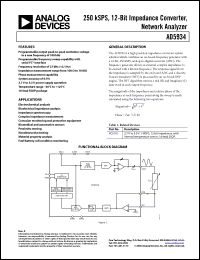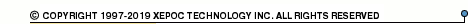More than12 555 330 queries processedPartname: EVAL-AD5934EB Description: 250 kSPS, 12-Bit Impedance Converter, Network Analyzer Manufacturer: Analog Devices Datasheet: PDF (513K).Click here to download *) The AD5934 is a high precision impedance converter system solution which combines an on-board frequency generator with a 12-bit, 250 kSPS, analog-to-digital converter (ADC). The frequency generator allows an external complex impedance to be excited with a known frequency. The response signal from the impedance is sampled by the on-board ADC and a discrete Fourier transform (DFT) is processed by an on-board DSP engine. The DFT algorithm returns a real (R) and imaginary (I) data-word at each output frequency. The magnitude of the impedance and relative phase of the impedance at each frequency point along the sweep is easily calculated using the following two equations: